# Combination

Aptitude

In this post we will be discussing about combinations. It is assumed that you have prior knowledge of permutations. If you don’t have then I would suggest you to through permutation as we will be discussion combinations along with permutations.

## What is Combination?

Each of the different selections or groups that can be formed out of the given number of things by taking some or all of them is called combinations.

Mathematically, if we have n elements then the total number of different selections or groups (i.e., combinations) that can be formed by taking r number of things at a time (r <= n) is nCr = n! / r! (n - r)!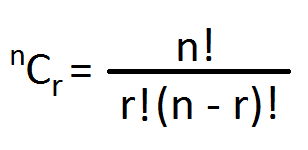Let’s take an example to under combinations in more detail.

Consider we have 3 persons A, B and C respectively and we have to form a committee of 2. So how many different committees of 2 persons may be formed from 3 persons.

So you see in this case we have to find the number of different groups (committees) that can be formed i.e., the combinations of 3 persons taken 2 at a time.

According to the problem we have 3 candidates and 2 posts. Now these two posts can be filled by the three persons A, B and C taken two at a time in the following ways:

AB

BC

CA

Using the combination formula we will get the same result. 3C2 = 3! / (2! X 1!) = 3

So we can have 3 different committees.

## Permutations and Combinations comparison

Let us now compare permutations and combinations using the above problem.

First we will take permutations.

The number of different arrangements that can be formed by taking 2 persons at a time out of 3 is 3P2 = 3! / 1! = 6

And the arrangements are as follows:

AB

AC

BA

BC

CA

CB

Now we will look at the combinations part.

The number of different groups that can be formed by taking 2 persons at a time out of 3 is 3C2 = 3! / (2! X 1!) = 3

And the combinations are as follows:

AB

BC

CA

Now why is it that we have 6 permutations and 3 combinations?

Well the answer to this question is very simple. Permutation is all about arrangements while on the other hand combination is all about selections.

So AB and BA are 2 different arrangements (permutation) but 1 single selection (combination).

## Relation between Permutation and Combination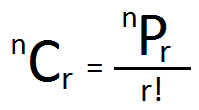Let’s take see some examples of combinations.

Q. How many different committees of 3 members may be formed from 4 men and 2 women?

A. According to the question, we need to find the number of combinations of 6 elements taken 3 at a time.

So 3 items can be selected out of 6 in 6C3 = 6! / (3! X 3!) = 20 ways.

Therefore the required combination is 20.

Q. A man has 6 friends. In how many ways can he invite one or more of them for dinner?

A. He can invite one or more friends by inviting 1 friend, or 2 friends or 3 friends, or all the 6 friends.

1 friend can be selected out of 6 in 6C1 = 6 ways

2 friends can be selected out of 6 in 6C2 = 15 ways

3 friends can be selected out of 6 in 6C3 = 20 ways

4 friends can be selected out of 6 in 6C4 = 15 ways

5 friends can be selected out of 6 in 6C5 = 6 ways

6 friends can be selected out of 6 in 6C6 = 1 ways

Therefore the required number of ways (combinations) = 6 + 15 + 20 + 15 + 6 + 1 = 63

Q. Find the number of diagonals that can be formed by joining the vertices of a hexagon?

A. Hexagon has 6 vertices and 6 sides. To find the number of diagonals we have to find the number of combinations of 6 vertices taken 2 at a time.

So 6C2 = 15

Now by joining 2 vertices we get 15 different lines. These 15 different lines include both the sides of the hexagon and the diagonals.

Hence the required number of diagonals = Total number of lines – Total number of sides = 15 – 6 = 9

Similarly,

Q. Find the total number of diagonals of an octagon?

A. Octagon has 8 sides and 8 vertices.

Total number of different lines (combinations) that can be formed of 8 vertices taken 2 at a time is 8C2 = 28

Therefore the required number of vertices = Total number of lines – Total number of sides = 28 – 8 = 20

## Handshakes

Q. If in a party everyone has shaken hands with everyone else, it was found that 66 handshakes were exchanged. If no two persons have shaken hands twice, find the number of persons present in the party?

A. This is a case of combinations.

Let the number of persons present in the party be n.

According to the question we need to find the combinations of n persons taken 2 at a time i.e., nC2.

And it is given that nC2 = 66

i.e., n! / (2! X (n-1)!) = 66

Or, n (n-1) = 132

Or, n (n-1) = 12 x 11

Or, n = 12

Therefore there were 12 persons present in the party.

## Red, White and Blue Balls

Q. A box contains 7 red, 6 white and 4 blue balls. How many selections of 3 balls can be made so that

i. all the three balls are red

ii. None are red

iii. There is one ball of each color

A. Total number of balls = 7 + 6 + 4 = 17

i. 3 red balls can be selected out of 7 in 7C3 = 35 ways.

ii. None of the 3 balls will be red if we select the 3 balls from 6 white and 4 blue balls.

So 3 balls can be selected out of 10 in 10C3 = 120 ways.

iii. 1 red ball can be selected out of 7 in 7C1 = 7 ways.

1 white ball can be selected out of 6 in 6C1 = 6 ways.

1 blue ball can be selected out of 4 in 4C1 = 4 ways.

Therefore the required number of selections of 1 red, 1 white and 1 blue ball = 7 x 6 x 4 = 168

Q. How many different words can be formed from 12 consonants and 5 vowels by taking 4 consonants and 3 vowels in each word?

A. 4 consonants can be selected out of 12 in 12C4 = 495 ways.

3 vowels can be selected out of 5 in 5C3 = 10 ways.

So, 4 consonants and 3 vowels can be selected in 495x10 = 4950 ways.

We have 7 letters and these 7 letters can be arranged (permutation) different in each word.

So the total number of different arrangements (permutations) of 7 letters by taking all at a time = 7P7 = 7! = 5040

Therefore the required number of words = 4950 x 5040 = 24948000.

## Repeated Elements

Q. In how many ways can 4 letters be selected from the word EXAMINATION?

A. There are 11 letters in the given word of which 5 are different and the following letters are repeated:

A – 2

I – 2

N – 2

So there are total 8 different kinds of letters viz. A, E, I, M, N, O, T and X.

We can select 4 letters in the following manner:

i. All the 4 letters are different.

ii. 2 are alike of one kind and the other 2 are alike of another kind. i.e., 2 set of pairs.

iii. 2 are alike of one kind and 2 are different. i.e., 1 pair and 2 different letters.

i. 4 different kinds of letters can be selected out of 8 in 8C4 = 70 ways.

ii. 2 set of alike letters can be selected out of 3 set of alike letters in 3C2 = 3 ways

iii. 1 pair can be selected out of 3 in 3C1 = 3 ways and 2 different letters can be selected out of 7 in 7C2 = 21 ways. So the required number of ways = 3 x 21 = 63

Therefore required total number of ways = 70 + 3 + 63 = 136

## Circular Problem

Q. In how many ways can 3 girls and 3 boys be seated at a round table, so that any 2 and only 2 of the girls are together?

A. According to the problem we have total 6 persons. Let the seats be numbered as 1, 2, 3, 4, 5 and 6 as shown in figure below.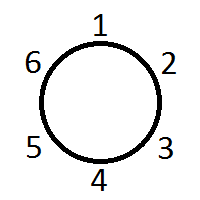Let any two girls occupy seat 1 and 3. Then the third girls can occupy seat no. 4 or 5 as only 2 girls can sit together. And the 3 boys can occupy the remaining 3 seats.

So we have to find three things:

Number of ways in which seat 1 and 2 are occupied by any two girls.

Number of ways in which seat 4 or 5 is occupied by the third girl.

Number of ways in which the remaining 3 seats are occupied by 3 boys.

Part 1

2 girls can be selected out of 3 in 3C2 = 3 ways.

Seat 1 and 2 can be occupied by any 2 girls in 2P2 = 2 ways.

So total number of ways possible = 3 x 2 = 6

Part 2

Number of ways in which the third girl can occupy any one of the two seats (4 or 5) = 2 ways.

Part 3

Number of ways in which the remaining 3 seat can be occupied by 3 boys = 3P3 = 6 ways.

Therefore required number of ways = 6 x 2 x 6 = 72

### Total number of combinations of n things not all different

Total number of combinations of n things of which x is alike of one kind, y is alike of second kind and z is alike of third kind is

{(x + 1) (y + 1) (z + 1)} – 1

## Division into 2 groups

There are (m + n) different elements and we have to divide it into 2 groups of size m and n.

m elements can be selected out of (m + n) different elements in (m + n)Cm ways. Once this is done the remaining n elements can be selected out of n in nCn i.e., 1 way.

Therefore the required number of ways in which (m + n) different elements can be divided into two groups containing m and n things respectively is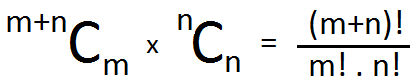If m = n, the two groups are equal and in this case the number of different ways of subdivision is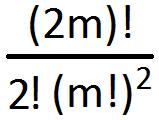If 2m things are distributed equally among two persons, then the number of ways is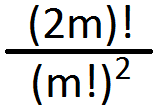## Division into 3 groups

There are (m + n + p) different elements and we have to divide it into 3 groups of size m, n and p.

m elements can be selected out of (m + n + p) different elements in (m + n + p)Cm ways. Once this is done n elements can be selected out of (n + p) in (n + p)Cn way and the remaining p elements can be selected out of p in pCp = 1 way.

Therefore the required number of ways in which (m + n + p) different elements can be divided into 3 groups containing m, n and p things respectively is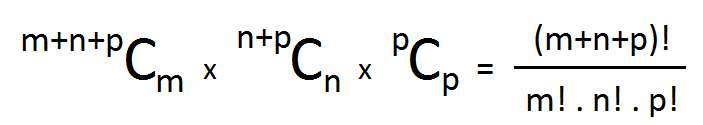If m = n = p, the three groups are equal and in this case the number of different ways of subdivision is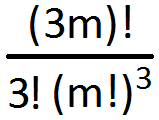If 3m things are distributed equally among three persons, then the number of ways is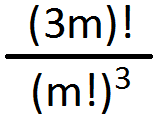Questions

Q. Find the number of ways in which 12 students can be distributed among 3 groups?

A. 12 students can be distributed among 3 groups in 12! / (3! X (4!)3) = 5775.

Q. Find the number of ways in which 12 apples can be distributed among 3 students?

A. The required number of ways in 12 apples can be distributed equally among 3 students = 12! / (3!)3 = 34650.